# python与tensorflow的关系_Tensorflow不是一个普通的Python库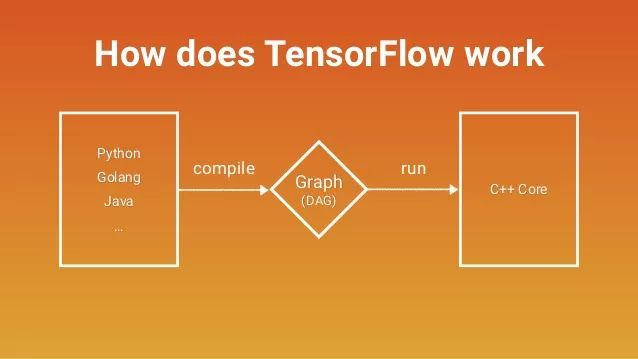Tensorflow不是一个普通的Python库

import tensorflow as tfimport tensorflow as tf

two_node = tf.constant(2)

print two_node

Tensor("Const:0", shape=(), dtype=int32)import tensorflow as tf

two_node = tf.constant(2)

another_two_node = tf.constant(2)

two_node = tf.constant(2)

tf.constant(3)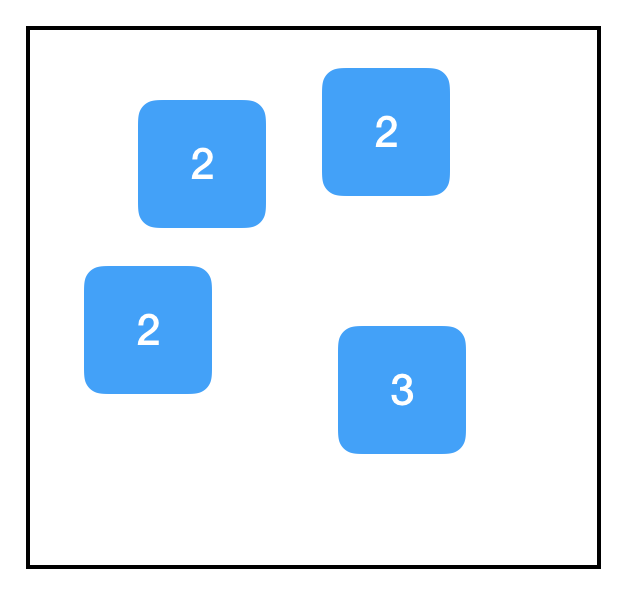import tensorflow as tf

two_node = tf.constant(2)

another_pointer_at_two_node = two_node

two_node = None

print two_node

print another_pointer_at_two_node

None

Tensor("Const:0", shape=(), dtype=int32)import tensorflow as tf

two_node = tf.constant(2)

three_node = tf.constant(3)

sum_node = two_node + three_node ## 相当于 tf.add(two_node, three_node)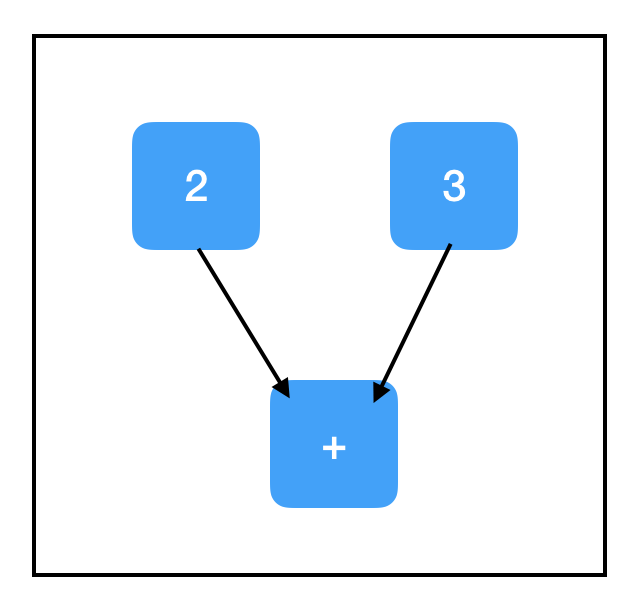import tensorflow as tf

two_node = tf.constant(2)

three_node = tf.constant(3)

sum_node = two_node + three_node

sess = tf.Session()

print sess.run(sum_node)

5import tensorflow as tf

two_node = tf.constant(2)

three_node = tf.constant(3)

sum_node = two_node + three_node

sess = tf.Session()

print sess.run([two_node, sum_node])

[2, 5]import tensorflow as tf

input_placeholder = tf.placeholder(tf.int32)

sess = tf.Session()

print sess.run(input_placeholder)

Traceback (most recent call last):

...

InvalidArgumentError (see above for traceback): You must feed a value for placeholder tensor 'Placeholder'with dtype int32

[[Node: Placeholder = Placeholder[dtype=DT_INT32, shape=, _device="/job:localhost/replica:0/task:0/device:CPU:0"]()]]...不是个好兆头。这是一个典型的失败案例，因为占位符本身没有初始值，再加上我们没有对它赋值，Tensorflow出现了个bug。

import tensorflow as tf

input_placeholder = tf.placeholder(tf.int32)

sess = tf.Session()

print sess.run(input_placeholder, feed_dict={input_placeholder: 2})

2import tensorflow as tf

input_placeholder = tf.placeholder(tf.int32)

three_node = tf.constant(3)

sum_node = input_placeholder + three_node

sess = tf.Session()

print sess.run(three_node)

print sess.run(sum_node)

3

Traceback (most recent call last):

...

InvalidArgumentError (see above for traceback): You must feed a value for placeholder tensor 'Placeholder_2'with dtype int32

[[Node: Placeholder_2 = Placeholder[dtype=DT_INT32, shape=, _device="/job:localhost/replica:0/task:0/device:CPU:0"]()]]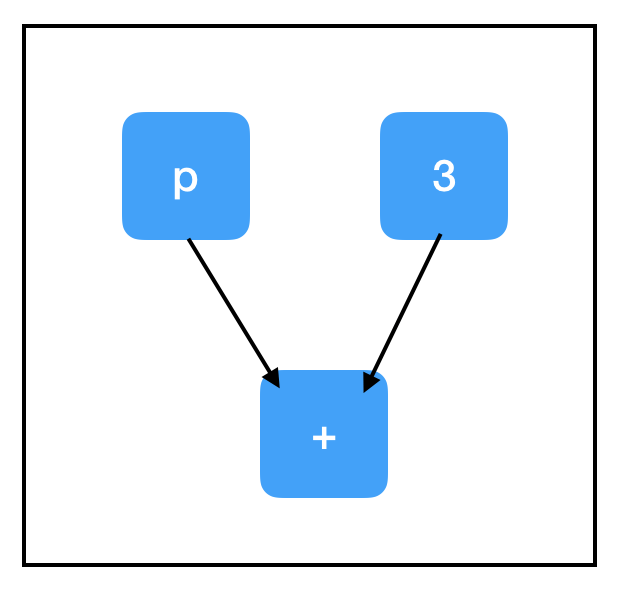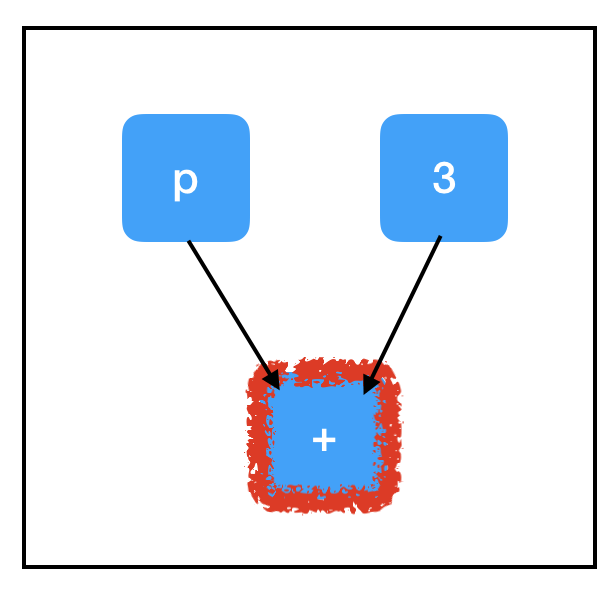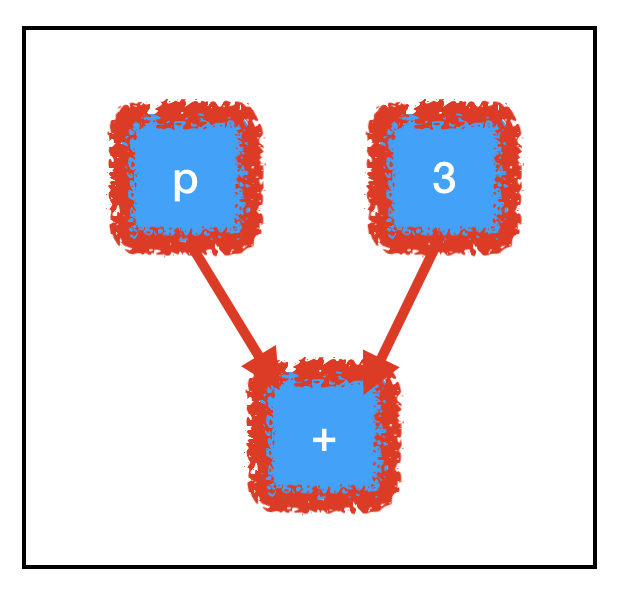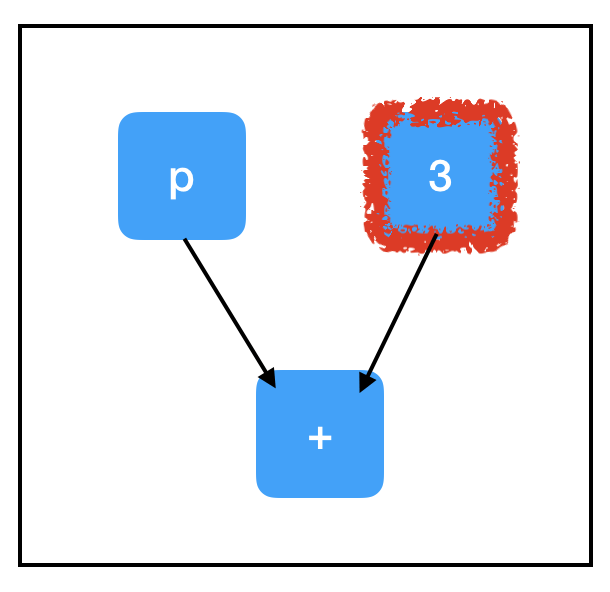Tensorflow的框架优势离不开计算路径设计。想象一下，如果我们手里有一幅巨型计算图，其中包含大量不必要的节点，通过这样的计算方式，我们可以绕过大多数点，只计算必要内容，这就为节省大量运行时间提供了可能性。此外，它还允许我们构建大型的“多用途”计算图，这些图中可以有一些共享的核心节点，但我们可以通过不同计算路径来进行不同的计算。

import tensorflow as tf

count_variable = tf.get_variable("count", [])

sess = tf.Session()

print sess.run(count_variable)

Traceback (most recent call last):

...

tensorflow.python.framework.errors_impl.FailedPreconditionError: Attempting to use uninitialized value count

[[Node: _retval_count_0_0 = _Retval[T=DT_FLOAT, index=0, _device="/job:localhost/replica:0/task:0/device:CPU:0"](count)]]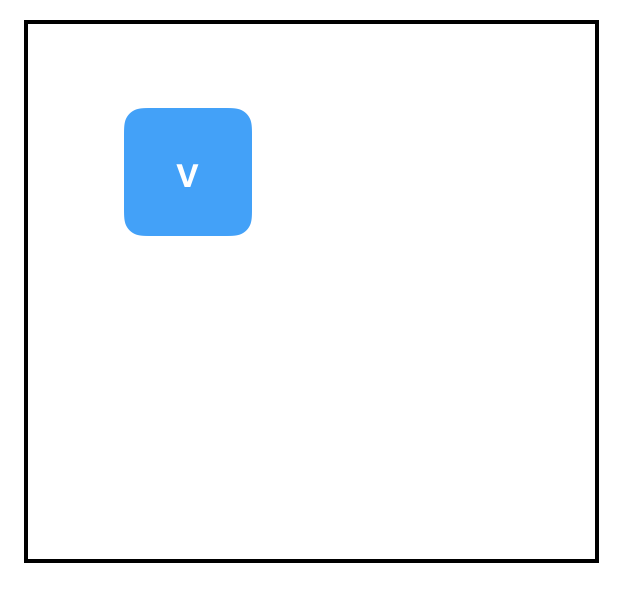import tensorflow as tf

count_variable = tf.get_variable("count", [])

zero_node = tf.constant(0.)

assign_node = tf.assign(count_variable, zero_node)

sess = tf.Session()

sess.run(assign_node)

print sess.run(count_variable)

0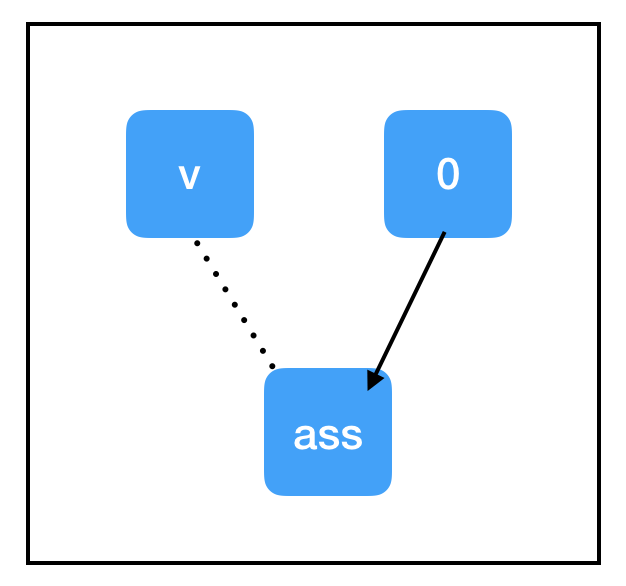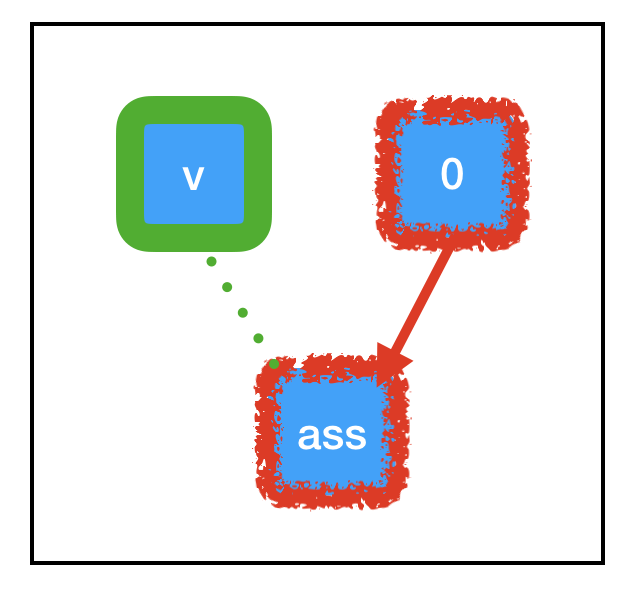import tensorflow as tf

const_init_node = tf.constant_initializer(0.)

count_variable = tf.get_variable("count", [], initializer=const_init_node)

sess = tf.Session()

print sess.run([count_variable])

Traceback (most recent call last):

...

tensorflow.python.framework.errors_impl.FailedPreconditionError: Attempting to use uninitialized value count

[[Node: _retval_count_0_0 = _Retval[T=DT_FLOAT, index=0, _device="/job:localhost/replica:0/task:0/device:CPU:0"](count)]]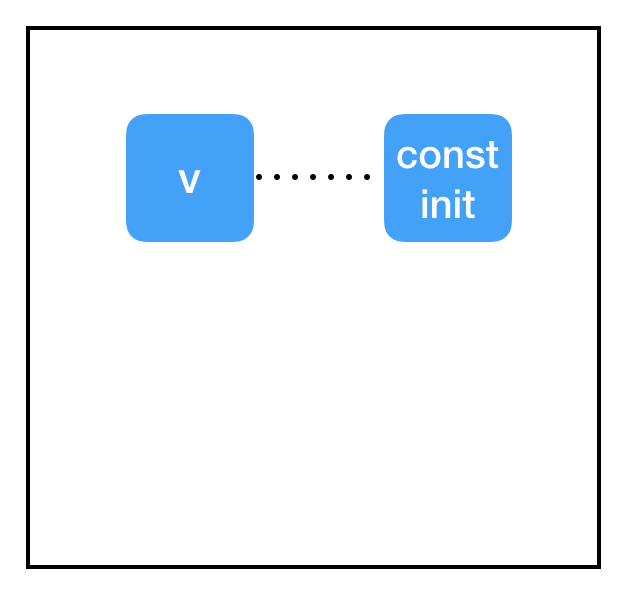import tensorflow as tf

const_init_node = tf.constant_initializer(0.)

count_variable = tf.get_variable("count", [], initializer=const_init_node)

init = tf.global_variables_initializer()

sess = tf.Session()

sess.run(init)

print sess.run(count_variable)

0.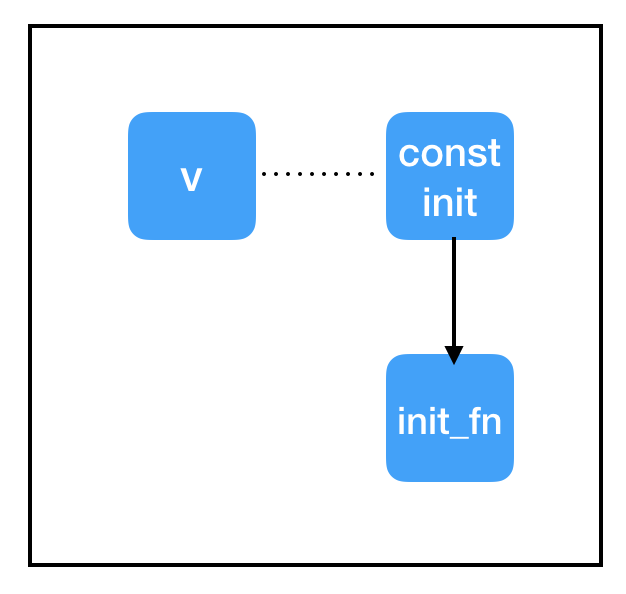02-03579
07-28970
11-282万+
05-112万+
08-106683
11-102695
11-24192
07-212万+
06-108152
06-052万+
11-082902
07-091294
04-111643
10-292459
04-073910
02-033210
12-031114
01-1565
11-26123
12-19765

### “相关推荐”对你有帮助么？

•非常没帮助
•没帮助
•一般
•有帮助
•非常有帮助被折叠的  条评论 为什么被折叠?到【灌水乐园】发言点击重新获取扫码支付1.余额是钱包充值的虚拟货币，按照1:1的比例进行支付金额的抵扣。
2.余额无法直接购买下载，可以购买VIP、C币套餐、付费专栏及课程。余额充值